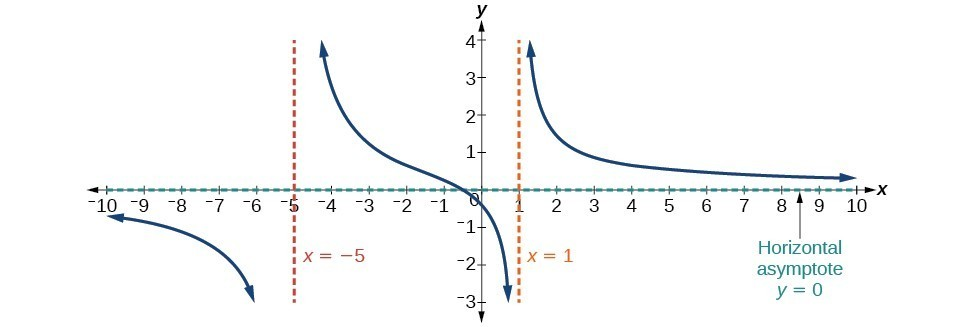## Horizontal Asymptotes and Intercepts

### Learning Outcomes

• Use the degree of the numerator and denominator of a rational function to determine what kind of horizontal asymptote it will have.
• Calculate slant asymptotes.
• Determine the intercepts of a rational function in factored form.

While vertical asymptotes describe the behavior of a graph as the output gets very large or very small, horizontal asymptotes help describe the behavior of a graph as the input gets very large or very small. Recall that a polynomial’s end behavior will mirror that of the leading term. Likewise, a rational function’s end behavior will mirror that of the ratio of the leading terms of the numerator and denominator functions.

There are three distinct outcomes when checking for horizontal asymptotes:

Case 1: If the degree of the denominator > degree of the numerator, there is a horizontal asymptote at $y=0$.

Example: $f\left(x\right)=\dfrac{4x+2}{{x}^{2}+4x - 5}$

In this case the end behavior is $f\left(x\right)\approx \frac{4x}{{x}^{2}}=\frac{4}{x}$. This tells us that, as the inputs increase or decrease without bound, this function will behave similarly to the function $g\left(x\right)=\frac{4}{x}$, and the outputs will approach zero, resulting in a horizontal asymptote at $y=0$. Note that this graph crosses the horizontal asymptote.Horizontal Asymptote $y=0$ when $f\left(x\right)=\dfrac{p\left(x\right)}{q\left(x\right)},q\left(x\right)\ne{0}\text{ where degree of }p<\text{degree of q}$.

Case 2: If the degree of the denominator < degree of the numerator by one, we get a slant asymptote.

Example: $f\left(x\right)=\dfrac{3{x}^{2}-2x+1}{x - 1}$

In this case the end behavior is $f\left(x\right)\approx \frac{3{x}^{2}}{x}=3x$. This tells us that as the inputs increase or decrease without bound, this function will behave similarly to the function $g\left(x\right)=3x$. As the inputs grow large, the outputs will grow and not level off, so this graph has no horizontal asymptote. However, the graph of $g\left(x\right)=3x$ looks like a diagonal line, and since $f$ will behave similarly to $g$, it will approach a line close to $y=3x$. This line is a slant asymptote (NOTE: the graph of the function itself is just a “sketch” within the parameters of the asymptotes).Slant Asymptote when $f\left(x\right)=\dfrac{p\left(x\right)}{q\left(x\right)},q\left(x\right)\ne 0$ where degree of $p>\text{ degree of }q\text{ by }1$.

To find the equation of the slant asymptote, divide $\dfrac{3{x}^{2}-2x+1}{x - 1}$. The quotient is $3x+1$, and the remainder is 2. The slant asymptote is the graph of the line $g\left(x\right)=3x+1$.

Case 3: If the degree of the denominator = degree of the numerator, there is a horizontal asymptote at $y=\frac{{a}_{n}}{{b}_{n}}$, where ${a}_{n}$ and ${b}_{n}$ are the leading coefficients of $p\left(x\right)$ and $q\left(x\right)$ for $f\left(x\right)=\frac{p\left(x\right)}{q\left(x\right)},q\left(x\right)\ne 0$.

Example: $f\left(x\right)=\dfrac{3{x}^{2}+2}{{x}^{2}+4x - 5}$

In this case the end behavior is $f\left(x\right)\approx \frac{3{x}^{2}}{{x}^{2}}=3$. This tells us that as the inputs grow large, this function will behave like the function $g\left(x\right)=3$, which is a horizontal line. As $x\to \pm \infty ,f\left(x\right)\to 3$, resulting in a horizontal asymptote at $y=3$. Note that this graph crosses the horizontal asymptote.Horizontal Asymptote when $f\left(x\right)=\frac{p\left(x\right)}{q\left(x\right)},q\left(x\right)\ne 0\text{ where degree of }p=\text{degree of }q$.

Notice that, while the graph of a rational function will never cross a vertical asymptote, the graph may or may not cross a horizontal or slant asymptote. Also, although the graph of a rational function may have many vertical asymptotes, the graph will have at most one horizontal (or slant) asymptote.

It should be noted that, if the degree of the numerator is larger than the degree of the denominator by more than one, the end behavior of the graph will mimic the behavior of the reduced end behavior fraction. For instance, if we had the function

$f\left(x\right)=\dfrac{3{x}^{5}-{x}^{2}}{x+3}$

with end behavior

$f\left(x\right)\approx \dfrac{3{x}^{5}}{x}=3{x}^{4}$,

the end behavior of the graph would look similar to that of an even polynomial with a positive leading coefficient.

As $x\to \pm \infty , f\left(x\right)\to \infty$

### A General Note: Horizontal Asymptotes of Rational Functions

The horizontal asymptote of a rational function can be determined by looking at the degrees of the numerator and denominator.

• Degree of numerator is less than degree of denominator: horizontal asymptote at
• $y=0$
• Degree of numerator is greater than degree of denominator by one: no horizontal asymptote; slant asymptote.
• Degree of numerator is equal to degree of denominator: horizontal asymptote at ratio of leading coefficients.

### Example: Identifying Horizontal and Slant Asymptotes

For the functions below, identify the horizontal or slant asymptote.

1. $g\left(x\right)=\dfrac{6{x}^{3}-10x}{2{x}^{3}+5{x}^{2}}$
2. $h\left(x\right)=\dfrac{{x}^{2}-4x+1}{x+2}$
3. $k\left(x\right)=\dfrac{{x}^{2}+4x}{{x}^{3}-8}$

### Try it

Watch this video to see more worked examples of determining which kind of horizontal asymptote a rational function will have.

### Example: Identifying Horizontal Asymptotes

In the sugar concentration problem earlier, we created the equation $C\left(t\right)=\dfrac{5+t}{100+10t}$.

Find the horizontal asymptote and interpret it in context of the problem.

### Example: Identifying Horizontal and Vertical Asymptotes

Find the horizontal and vertical asymptotes of the function

$f\left(x\right)=\dfrac{\left(x - 2\right)\left(x+3\right)}{\left(x - 1\right)\left(x+2\right)\left(x - 5\right)}$

### Try It

Find the vertical and horizontal asymptotes of the function:

$f\left(x\right)=\dfrac{\left(2x - 1\right)\left(2x+1\right)}{\left(x - 2\right)\left(x+3\right)}$

### A General Note: Intercepts of Rational Functions

A rational function will have a y-intercept when the input is zero, if the function is defined at zero. A rational function will not have a $y$-intercept if the function is not defined at zero.

Likewise, a rational function will have $x$-intercepts at the inputs that cause the output to be zero. Since a fraction is only equal to zero when the numerator is zero, $x$-intercepts can only occur when the numerator of the rational function is equal to zero.

### Example: Finding the Intercepts of a Rational Function

Find the intercepts of $f\left(x\right)=\dfrac{\left(x - 2\right)\left(x+3\right)}{\left(x - 1\right)\left(x+2\right)\left(x - 5\right)}$.

### Try It

Given the reciprocal squared function that is shifted right 3 units and down 4 units, write this as a rational function. Then, find the $x$– and $y$-intercepts and the horizontal and vertical asymptotes.

Watch the following video to see more worked examples of finding asymptotes, intercepts and holes of rational functions.

## Contribute!

Did you have an idea for improving this content? We’d love your input.